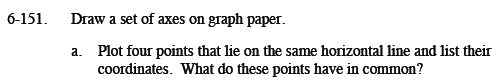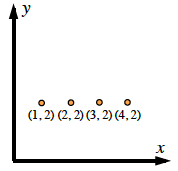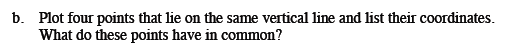### Home > MC1 > Chapter 6 > Lesson 6.3.3 > Problem6-151

6-151.
1. . Draw a set of axes on graph paper. Homework Help ✎

1. Plot four points that lie on the same horizontal line and list their coordinates. What do these points have in common?

2. Plot four points that lie on the same vertical line and list their coordinates. What do these points have in common?To the right is one example of how to draw a graph for this problem. What do these points share?Draw a graph similar to the one in part (a), but align the points vertically rather than horizontally.
Which coordinate do they have in common?